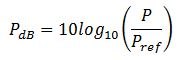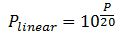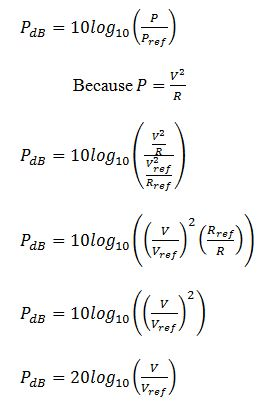# The Decibel

Posted by in on 3-4-13

The decibel (dB) is one of the most widely used measurement units in electrical engineering, but is a common source of misunderstanding for unfamiliar engineers. Fundamentally, the dB is a ratio, but the concept the decibel is also used to describe absolute quantities, of which power is the most common example.

1. Definition of the Decibel
2. When to Use 10log10 or 20log10
3. Why Engineers Use the Decibel
4. Common Decibel Units Used in Electrical Engineering
• dB
• dBm
• dBW
• dBc
• dBa
• dBi

## Definition of the Decibel

The decibel is a unit of measure that quantifies the ratio of two powers using the logarithmic scale. The decibel is mathematically defined:where P_ref is the reference power and P is the power to be quantified. The dB is positive when describing powers greater than the reference power and negative for powers less than the reference power.

The decibel (dB) is unitless, just like when we talk about ratios on a linear scale (e.g. 2x or 10:1).

The dB can be converted from the logarithmic scale back to linear using the following equation:for power quantities, andfor field quantities.

The dB is used to describe relative powers, or ratios, but there are also many derived units that represent absolute quantities or reference specific quantities . These units are denoted with another letter after the dB, such as dBm, dBW, etc.

## When to Use 10log10 and 20log10

Keep in mind that the decibel represents the ratio between to power quantities. When the two ratio quantities are already powers, the previous equation can be used (shown again here):When the two ratio quantities are field quantities (or amplitudes) use the definition:The conversion to dB using 20log10 is required because squared field quantities (like voltage and current) are proportional to power. This is demonstrated using the ratio of two voltages:The only assumption used is that the two resistances are the same for both voltages, which is a good assumption for well-designed circuits.

## Why Engineers Use dB

Engineers use the decibel (dB) system because it’s a convenient way of manipulating and visualizing large numbers and large dynamic ranges.

It can be difficult and cumbersome to talk about a receiver that has, for example, 1.4125 million times the amplification of the received input signal. It’s easier to say the the amplifier has 61.5 dB of gain.

Logarithms can be added instead of multiplied, so it’s more manageable to understand gain stages in terms of dB than linear scales. For example, what if I added another gain stage of 1.22 to the previous example. I could either multiply 1.4125e6 by 1.22 in linear scale, or add 0.5 dB to 61.5 dB for the same gain description. Adding small numbers is much easier than multiplying large numbers.

Another advantage to using the dB scale is the ease of visualization of signals with large dynamic ranges. The following figures illustrate this point using a noise floor and two sinusoids.

Both plots are based on the same data. The linear plot shows the sinusoid at 18 MHz, but we can visualize nothing about the floor or any other signals in the spectrum. On the other hand, the plot in dB clearly shows the relative amplitudes of both the sinusoids and their proximity to the noise floor.

## Common Decibel Units

dB

The dB is the fundamental unit of logarithmic power ratios. Powers directly use the equation:while field or amplitude quantities relate to power through the square and use the equation:dBm
An absolute power unit that references 1 mW. The mW is a power unit, so 10log10 is used to convert from linear.

dBW
An absolute power unit that references 1 W. The W is a power unit, so 10log10 is used to convert from linear.

dBc
An relative power unit that references the power of a carrier. The carrier power is a power unit, so 10log10 is used to convert from linear.

The dBc is typically used in communication to denote signals of interest with respect to the carrier sinusoid.

dBa
A relative power unit that references the power of an input signal. The input signal power is a power unit, so 10log10 is used to convert from linear.

The dBa is typically used to specify or quantify filter performance. For example, a passband may be specified at 0.5 dBa of loss with a stopband loss of more than 40 dBa.

dBi
A relative antenna field unit that references an isotropically radiating field. The antenna field is a field unit, so 20log10 is used to convert from linear.

The dBi is typically used to describe the directivity of antennas. Antenna fields are compared to an isotropic source, which is an ideal source that emits electromagnetic energy equally in all three dimensions.

There are many other units derived from the dB, but these are the most typically used units in electrical engineering.Did you enjoy this article?